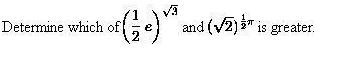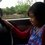# which is the greater? ( i still dont understand )without calculatorNote by NurFitri Hartina
6 years, 3 months ago

This discussion board is a place to discuss our Daily Challenges and the math and science related to those challenges. Explanations are more than just a solution — they should explain the steps and thinking strategies that you used to obtain the solution. Comments should further the discussion of math and science.

When posting on Brilliant:

• Use the emojis to react to an explanation, whether you're congratulating a job well done , or just really confused .
• Ask specific questions about the challenge or the steps in somebody's explanation. Well-posed questions can add a lot to the discussion, but posting "I don't understand!" doesn't help anyone.
• Try to contribute something new to the discussion, whether it is an extension, generalization or other idea related to the challenge.

MarkdownAppears as
*italics* or _italics_ italics
**bold** or __bold__ bold
- bulleted- list
• bulleted
• list
1. numbered2. list
1. numbered
2. list
Note: you must add a full line of space before and after lists for them to show up correctly
paragraph 1paragraph 2

paragraph 1

paragraph 2

[example link](https://brilliant.org)example link
> This is a quote
This is a quote
    # I indented these lines
# 4 spaces, and now they show
# up as a code block.

print "hello world"
# I indented these lines
# 4 spaces, and now they show
# up as a code block.

print "hello world"
MathAppears as
Remember to wrap math in $$ ... $$ or $ ... $ to ensure proper formatting.
2 \times 3 $2 \times 3$
2^{34} $2^{34}$
a_{i-1} $a_{i-1}$
\frac{2}{3} $\frac{2}{3}$
\sqrt{2} $\sqrt{2}$
\sum_{i=1}^3 $\sum_{i=1}^3$
\sin \theta $\sin \theta$
\boxed{123} $\boxed{123}$

Sort by:

$\LARGE \ln {2} \approx \frac {2}{3 - \frac {1^2}{9 - \frac {2^2}{15 - \frac {3^2}{21} }}} = \frac {445}{642} > 0.693$

Because $\ln{2} > 0.693 \Rightarrow ( \ln{2} )^2 > 0.48 = 3 \cdot \frac {4}{25} \Rightarrow \ln{2} > \frac {2\sqrt3}{5}$

And because $\pi > \frac {22}{7} - \frac {1}{630} > 3.1$

Now suppose that

$( \large \frac {e}{2} )^{\sqrt{3}} \geq (\sqrt2)^{\frac {\pi}{2} }$, raise both sides to the power of $4$

$\Rightarrow ( \large \frac {e}{2} )^{4 \sqrt{3}} \geq 2^{\pi}$, log both sides

$\large \Rightarrow 4 \sqrt{3} \space (1 - \ln 2) \geq \pi \space \ln 2$

$\large \Rightarrow 4 \sqrt{3} \geq \ln {2} \space ( \pi + 4\sqrt{3} )$

$\large \Rightarrow \frac { 4 \sqrt{3} }{ \pi + 4\sqrt{3} } \geq \ln {2}$

$\large \Rightarrow \frac { 4 \sqrt{3} }{ \pi + 4\sqrt{3} } \geq \ln {2} > \frac {2\sqrt3}{5}$

$\large \Rightarrow 10 - \pi > 4\sqrt{3}$

$\large \Rightarrow 10 - 3.1 > 10 - \pi > 4\sqrt{3}$, because $\pi > 3.1$

$\large \Rightarrow 6.9^2 > 48$, which is a contradiction.

Hence $\LARGE ( \frac {e}{2} )^{\sqrt{3}} < (\sqrt2)^{\frac {\pi}{2} }$

- 6 years, 3 months ago

Those are some heavy-duty bounds! I like the integral trick to get $\pi > 22/7$, and I see now why I was having trouble getting $\ln 2 > .693$. Where in the world does that crazy continued fraction expansion come from? :-)

- 6 years, 3 months ago

Scroll down to

$\LARGE \log (1 + \frac {x}{y} ) = \frac { 2} { 2y + x - \frac {(1x)^2}{3(2y+x) - \frac {(2x)^2}{5(2y+x) - \frac {(3x)^2}{7(2y+x) - \ldots } } } }$

Substitute $\large x = y = 1$

- 6 years, 3 months ago

that was very good, that's a lot of story

- 6 years, 3 months ago

We will show that the latter is the greater, some easy approximations will do the trick. Note that $1.57 < \pi / 2$, $\sqrt{3} < 1.74$, and $0.69 < \log 2$. So we want to show that $(e/2)^{174/100} < \sqrt{2}^{157/100}$, or $e^{174} < 2^{157/2 + 174}$, meaning that $174 < \frac{505}{2} \log 2$. Since $0.69 < \log 2$, we have that $174 < 174.225$, which is true.

- 6 years, 3 months ago

(meant to be a reply to George W.'s comment) I think your last step is off since you went $174<\frac{505}{2}\log 2 < (.69)\frac{505}{2}$, which is backwards. However, if you use $\log 2 < .7$ (which entails first showing that $e^7 > 1024$ from the definition of $e$ (using memorized values is kinda cheaty)) then you get $174 < 176.75$.

Overall, I think a different approach is called for since showing $3.14 < \pi$ by hand seems daunting in itself. Getting $\sqrt{3} < 1.74$ is easy to show once you have it conjectured, but Newton's method is pretty fast by hand if you wanna be a bit more hardcore. All this is beside the point!

This kind of problem is usually solved by comparing to a third number that's between them. Maybe $\sqrt{5}$? I thought about expanding $\pi/4 = 1-\frac{1}{3} + \frac{1}{5}...$, but that convergence is too slow to be helpful.

- 6 years, 3 months ago

Actually, $0.69 < \log 2 \approx 0.6931$, so $0.69 \frac{505}{2} < \frac{505}{2} \log 2$. Yeah, the memorized value is sort of cheating (although I find it easier to believe than memorizing partial fraction representations, but that's my opinion.) I'll see if I can find a nicer proof which doesn't require knowing $\log 2$, although I believe knowing $\pi$ is quite reasonable.

- 6 years, 3 months ago

Wow. Which level is this?

- 6 years, 3 months ago

Let, (e/2)^√3=a×(√2)^π/2

⇒e^√3×2^-√3=a×2^π/4

⇒e^√3=a×2^(π/4+√3)

⇒√3=lna+(π/4+√3)ln2 [ln]

⇒1.73205=lna+(3.1415926536/4+1.73205)×.6931 [ ln2≈2/[3−1^2/{9−2^2/(15−3^2/21)}]=445/642=.69314]

⇒lna=1.73205-1.744843

⇒lna=-.012793

⇒a=1/e^.012793

⇒1/e^.012793<1

so,(e/2)^√3 / (√2)^π/2 <1

so,(e/2)^√3<(√2)^π/2

- 6 years, 3 months ago

Use logarithms!

- 6 years, 3 months ago

Note that: (1/2e)^3/2=(1/2e)^3^1/2 and (2^1/2)^1/2pi=(2^1/2)^pi^1/2.

Since we are comparing those numbers, we can delete the latter " ^1/2 ". So we got (1/2e)^3 and (2^1/2)^pi.

1/2e ~ 1.36 ; 2^1/2 ~ 1.41 and pi > 3. So, (2^1/2)1/2pi is the greatest.

- 6 years, 3 months ago

The first one is (e/2)^(3^1/2). The second is ((2^1/2)^pi)^1/2 You can't remove the ^1/2 at the end of them. (Removing them involves squaring both sides, which gives (e/2)^(2*3^1/2) and (2^1/2)^pi)

- 6 years, 3 months ago

- 6 years, 3 months ago

Sorry dude, but I think you comitted a mistake. I do can remove them, because if we square, for example, (e/2)^(3^1/2), we get: [(e/2)^(3^1/2)]^2= (e/2)^2.3.(1/2)=(e/2)^3 . I used a identity from exponenciation, as you can see at http://en.wikipedia.org/wiki/Exponentiation#Identitiesandproperties

- 6 years, 3 months ago

The identity is $(a^b)^c = a^{bc}$, not $a^{b^c} = a^{bc}$. When you do $\left( \left( \dfrac{e}{2} \right)^{3^{1/2}} \right)^2 = \left( \dfrac{e}{2} \right)^{2 \cdot 3 \cdot 1/2}$, you accidentally made the mistake with $3^{1/2}$. (The result should have been $\left( \dfrac{e}{2} \right)^{2 \cdot (3^{1/2})}$; note that the 1/2 is as an exponent of the 3 instead of a multiplicand.)

- 6 years, 3 months ago

Ohh I see. Thanks Ivan for correct me, but I think that there is a solution similar to mine, because it's not necessary to go crazy with logarithms in this problem. I also have to ask for apologies to Suhail: Sorry dude, my bad.

- 6 years, 3 months ago

this is just sort of an easy mathematical task. why make things complicated? We are now in the 21st century (computer era).

- 6 years, 3 months ago